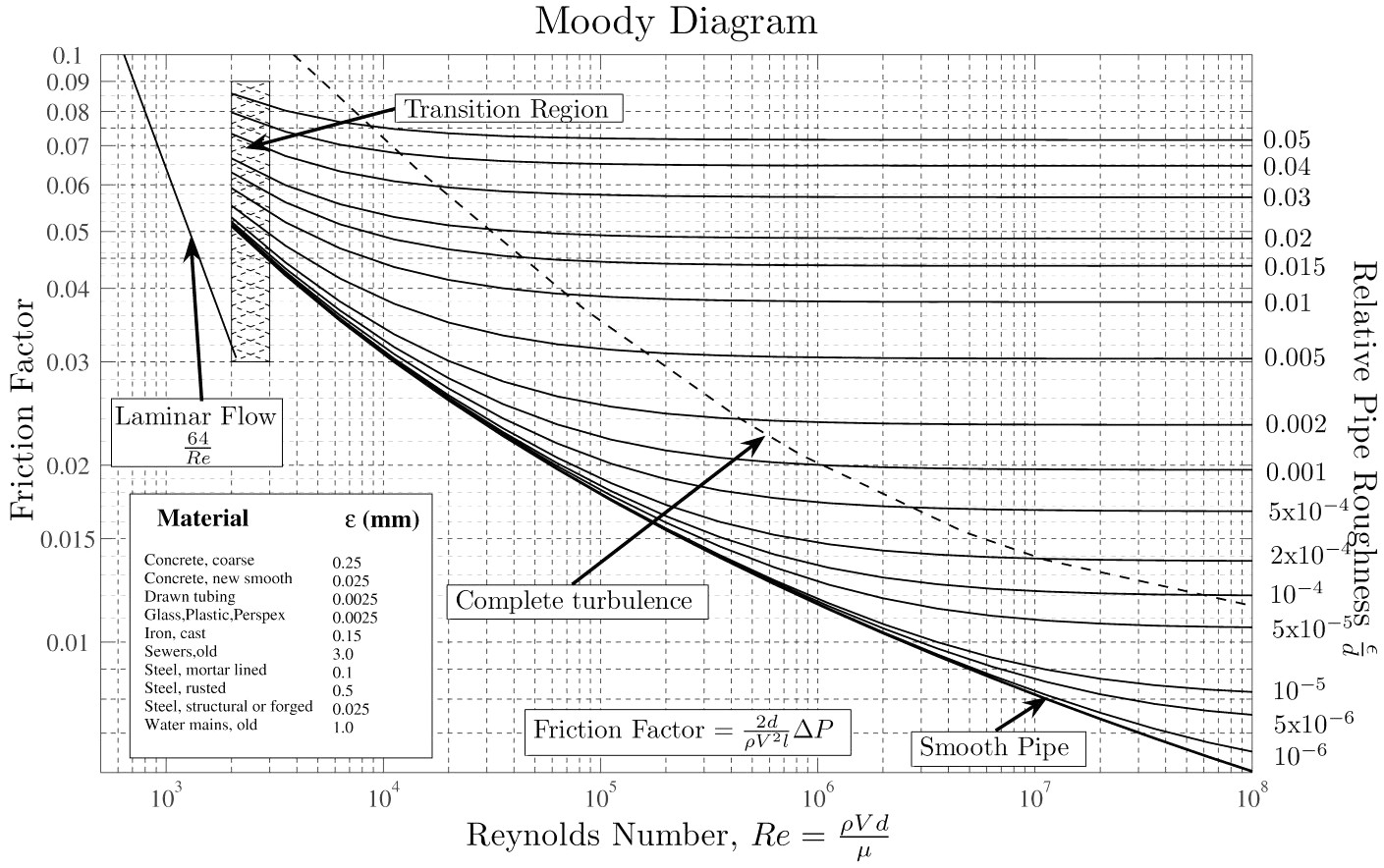# HOMER Pro 3.11Type: Input Variable Units: % Symbol: fh

The pipe head loss is the frictional loss in the hydro pipeline, expressed as a fraction of the available head.

Water (like any viscous fluid) flowing through a pipe experiences a loss in pressure due to friction. We can express this pressure loss in terms of a loss of head, where head is the vertical drop through which the fluid flows. In HOMER, you specify the pipe head loss as a percentage of the available head.

Small high-head, low-flow hydro systems typically experience pipe head losses of between 10% and 20%. With low-head systems, pipe head losses are typically only a few percent.

The head loss percentage is defined in terms of the absolute head loss hl and the total available head h: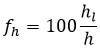The Darcy–Weisbach equation can be used to predict frictional losses in a circular pipe: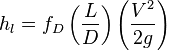where: hl = Absolute head loss due to friction, given in units of length fD = Darcy friction factor L = Pipe length D = Pipe diameter V = Flow velocity (where Q-dot is volumetric flow rate):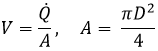g = Gravitational acceleration (i.e. 9.81 m/s2)

The Darcy friction factor fD can be calculated several different ways, including the well-known Moody diagram (below) or one of many on-line calculators. For laminar flows (Reynolds number, Re, less than 2300), you can use the following:

fD = 64/Re

The friction factor can vary for transition flows (2300 < Re < 4000), and a number of correlations are possible. The Moody diagram provides a good estimate in this method. For turbulent flows, the Moody diagram is a good reference, or you can compute fD by solving the Colebrook–White equation: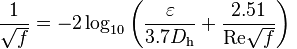where: ? = Roughness height Dh = Hydraulic diameter (inside diameter for circular tubes)